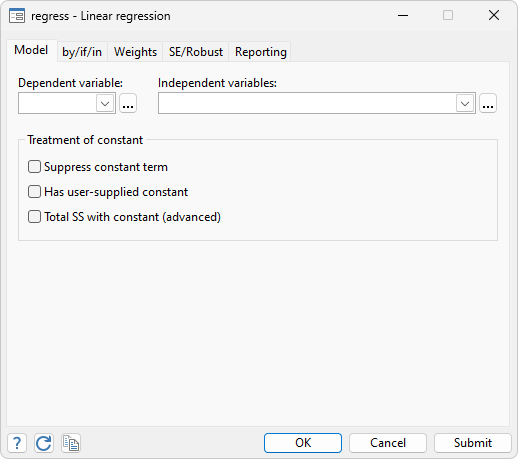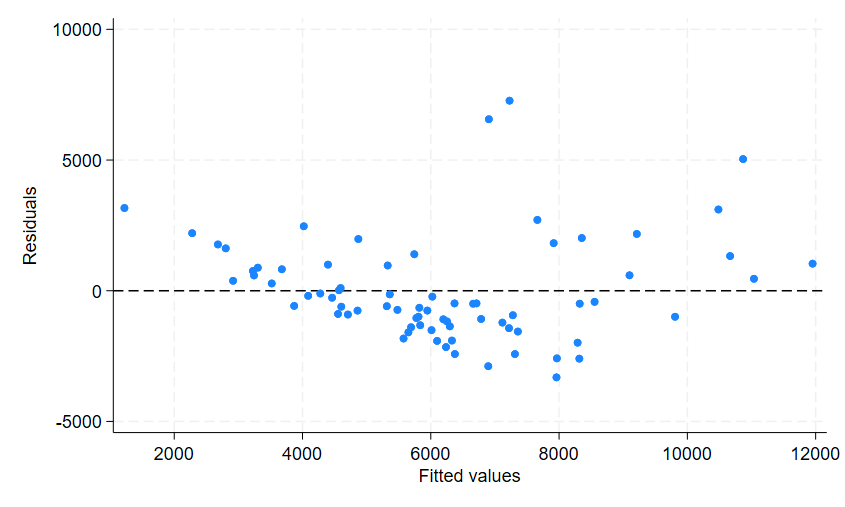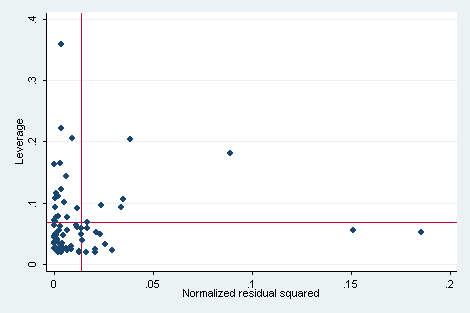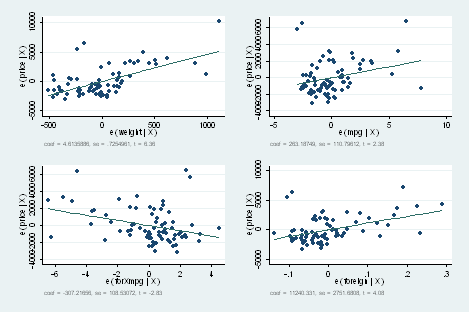»  Home »  Products »  Features »  Linear models »  Linear regression and influence

## Linear regression and influence

 Ramsey regression specification error test for omitted variables Cook and Weisberg test for heteroskedasticity Variance-inflation factors Cook’s distance COVRATIO DFBETAs DFITs Diagonal elements of hat matrix Residuals, standardized residuals, studentized residuals Standard errors of the forecast, prediction, and residuals Welsch distanceUnder the heading least squares, Stata can fit ordinary regression models, instrumental-variables models, constrained linear regression, nonlinear least squares, and two-stage least-squares models. (Stata can also fit quantile regression models, which include median regression or minimization of the absolute sums of the residuals.)

After fitting a linear regression model, Stata can calculate predictions, residuals, standardized residuals, and studentized (jackknifed) residuals; the standard error of the forecast, prediction, and residuals; the influence measures Cook’s distance, COVRATIO, DFBETAs, DFITS, leverage, and Welsch’s distance; variance-inflation factors; specification tests; and tests for heteroskedasticity.

Among the fit diagnostic tools are added-variable plots (also known as partial-regression leverage plots, partial regression plots, or adjusted partial residual plots), component-plus-residual plots (also known as augmented partial residual plots), leverage-versus-squared-residual plots (or L-R plots), residual-versus-fitted plots, and residual-versus-predictor plots (or independent variable plots). Each tool is available by typing one command.

For example, let’s start with a dataset that contains the price, weight, mpg, and origin (foreign or U.S.) for 74 cars:

. webuse auto
(1978 automobile data)

. regress price weight foreign##c.mpg

Source         SS       df       MS           Number of obs =      74
F(4, 69)      =   21.22
Model     350319665     4  87579916.3           Prob > F      =  0.0000
Residual     284745731    69  4126749.72           R-squared     =  0.5516
Total     635065396    73  8699525.97           Root MSE      =  2031.4

price   Coefficient  Std. err.      t    P>|t|     [95% conf. interval]

weight     4.613589   .7254961     6.36   0.000     3.166263    6.060914

foreign
Foreign      11240.33   2751.681     4.08   0.000     5750.878    16729.78
mpg     263.1875   110.7961     2.38   0.020     42.15527    484.2197

foreign#c.mpg
Foreign     -307.2166   108.5307    -2.83   0.006    -523.7294   -90.70368

_cons    -14449.58    4425.72    -3.26   0.002    -23278.65    -5620.51



We have used factor variables in the above example. The term foreign##c.mpg specifies to include a full factorial of the variables—main effects for each variable and an interaction. The c. just says that mpg is continuous. regress is Stata’s linear regression command. All estimation commands have the same syntax: the name of the dependent variable followed by the names of the independent variables. After estimation, we can review diagnostic plots:

. rvfplot, yline(0)Typing rvfplot displays a residual-versus-fitted plot, although we created the graph above by typing rvfplot, yline(0); this drew a line across the graph at 0. That you can discern a pattern indicates that our model has problems.

We obtain a leverage plot by typing:

. lvr2plotavplot draws added-variable plots, both for variables currently in the model and variables not yet in the model:

. avplot mpgAdded-variable plots are so useful that they are worth reviewing for every variable in the model:

. avplotsThe graph above is one Stata image and was created by typing avplots. The combined graph is useful because we have only four variables in our model, although Stata would draw the graph even if we had 798 variables in our model. The individual graphs would, however, be too small to be useful. That is why there is an avplot command.

Exploring the influence of observations in other ways is equally easy. For instance, we could obtain a new variable called cook containing Cook’s distance and then list suspicious observations by typing

. predict cook, cooksd, if e(sample)

. predict e if e(sample), resid

. list make price e cook if cook>4/74

make                price           e       cook

13.  Cad. Seville       15,906    5036.348   .3328515
24.  Ford Fiesta         4,389    3164.872   .0638815
28.  Linc. Versailles   13,466    6560.912   .1308004
42.  Plym. Arrow         4,647   -3312.968   .1700736



We could obtain all the DFBETAs and then list the four observations having the most negative influence on the foreign coefficient and the four observations having the most positive influence by typing

. dfbeta
Generating DFBETA variables ...

_dfbeta_1: DFBETA weight
_dfbeta_2: DFBETA 1.foreign
_dfbeta_3: DFBETA mpg
_dfbeta_4: DFBETA 1.foreign#c.mpg

. sort _dfbeta_2

. list make price foreign _dfbeta_2 in 1/4

make                price    foreign   _dfbeta_2

1.  Plym. Arrow         4,647   Domestic   -.6622424
3.  Linc. Versailles   13,466   Domestic   -.5283729
4.  Toyota Corona       5,719    Foreign    -.256431

. list make price foreign _dfbeta_2 in -4/l

make            price    foreign   _dfbet~2

71.  Volvo 260      11,995    Foreign   .2318289
72.  Plym. Champ     4,425   Domestic   .2371104
73.  Peugeot 604    12,990    Foreign   .2552032
74.  Cad. Seville   15,906   Domestic   .8243419